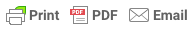Sorry, you do not have permission to ask a question, You must login to ask question. Become VIP Member

The flow of liquid through a channel with a free surface is defined as open channel flow. This free surface of the liquid is subjected to atmospheric pressure. Hence, open channel flow can also be defined as the flow of liquid through a passage at atmospheric pressure. The flow in an open channel takes place due to gravity that is achieved by providing a bed slope.

The flow of liquid through the open channel can be of several types like steady and unsteady flow, laminar or turbulent flow or uniform or non-uniform flow and finally sub-critical, critical and supercritical flow.

## Types of Flow in Open Channels

As mentioned above, the flow in the channel can be of:

In an open channel flow, if the flow parameters such as depth of flow, the velocity of flow and the rate of flow at a particular point on the fluid do not change with respect to time, then it is called as steady flow.

If v is the velocity of the fluid, Q is the rate of flow and d is the depth of flow, then for a steady flow:

dv/dt = 0; dQ/dt = 0; dy/dt = 0;

And is at any point on the open channel flow, the flow parameters like depth of flow, the velocity of flow and rate of flow do change their value with respect to time, then it is called as an unsteady flow. It is hence given by :

dv/dt ,dQ/dt and dy/dt not equal to Zero& Ad Free!

GET VIP MEMBERSHIP NOW!

### 2. Uniform Flow and Non-Uniform Flow

The flow in the channel is said to be uniform, if, for a given length of the channel, the velocity of flow, the depth of flow remains constant. i.e.

dy/dS = 0 ; dv/dS=0;

In a Non-uniform flow, the flow parameters like velocity, depth of flow, etc do not remain constant for a given length of the channel.

dy/dS and dv/dS not equal to zero

The Non-uniform flow can be again defined as Rapidly varying flow (R.V.F) and Gradually Varied Flow (G.V.F). In the case of R.V.F, the depth of flow rapidly changes over a smaller length of the channel. It rises up suddenly for a short length and settles back. While in a G.V.F, the depth of flow changes gradually over a longer length of the channel.

### 3. Laminar Flow and Turbulent Flow

Laminar and turbulent flow in open channel flow is defined based on the Reynolds Number, Re. The Reynolds number is given by the relation:

If the Reynolds number Re is less than 500 or 600, then the flow is called laminar flow. If the Reynolds number is more than 2000, then the flow is said to be turbulent.

A flow that has Reynolds number between 500 and 2000 is said to be in the transition state.

## 4. Critical, Sub-Critical and Super – Critical Flow

The open channel flow is categorized as critical or sub-critical or super-critical based on the Froude number Fe. Froude number is given by the relation:

Open channel flow is Sub-critical if the Froude number is less than 1. Sub-Critical open channel flow is also defined as a tranquil or streaming flow.

An open channel flow with a Froude number equal to one is a critical flow. And super-critical flow in open channel has a Froude number greater than 1. A supercritical flow is also termed as rapid flow or torrential flow or shooting flow.